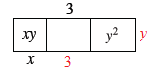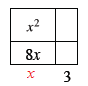### Home > CCA > Chapter 6 > Lesson 6.1.3 > Problem6-27

6-27.

For each generic rectangle fill in the missing parts. Write an equation showing the area as a sum equals the area as a product. Homework Help ✎

1.  $+3$ $xy$ $y^2$ $x$
• The product of the dimensions of the rectangle is equal to the area.

•• y(x + 3 + y) = xy + 3y + y2

1.  $x^2$ $8x$ $+3$
• Follow the same step as (a).

•